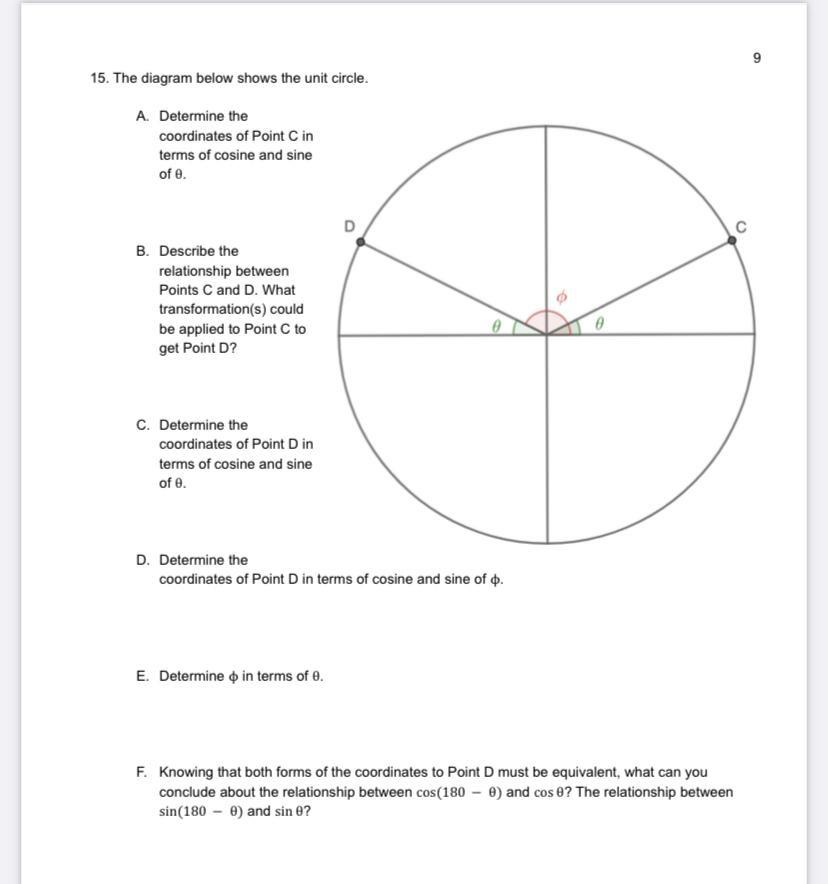Question:

# 9 15. The diagram below shows the unit circle. A. Determine the coordinates of Point C in terms of cosine and sine of e. D C B.9 15. The diagram below shows the unit circle. A. Determine the coordinates of Point C in terms of cosine and sine of e. D C B. Describe the relationship between Points C and D. What transformation(s) could be applied to Point C to get Point D? C. Determine the coordinates of Point Din terms of cosine and sine of e. D. Determine the coordinates of Point D in terms of cosine and sine of . E. Determine o in terms of e. F. Knowing that both forms of the coordinates to Point D must be equivalent, what can you conclude about the relationship between cos(180 - 0) and cose? The relationship between sin(180 - 0) and sine?